• 增量型pid
2020-09-02 15:07:36
struct _pid{
float SetSpeed;//定义设定值
float ActualSpeed;//定义实际值
float err;//定义偏差值
float err_next;//定义上一个偏差值
float err_last;//定义最上前的偏差值
float Kp, Ki, Kd;//定义比例、积分、微分系数
}pid;

{初始化}

void PID_init(){
pid.SetSpeed = 0.0;
pid.ActualSpeed = 0.0;
pid.err = 0.0;
pid.err_last = 0.0;
pid.err_next = 0.0;
//可设置下面的值，从而达到最佳效果
pid.Kp = 0.2;
pid.Ki = 0.04;
pid.Kd = 0.2;
}
{控制算法}

float PID_realize(float speed){
float incrementSpeed;
pid.SetSpeed = speed;
pid.err = pid.SetSpeed - pid.ActualSpeed;
incrementSpeed = pid.Kp*(pid.err - pid.err_next) + pid.Ki*pid.err + pid.Kd*(pid.err - 2 * pid.err_next + pid.err_last);//计算出增量
pid.ActualSpeed += incrementSpeed;
pid.err_last = pid.err_next;
pid.err_next = pid.err;
return pid.ActualSpeed;
}


更多相关内容
• 简易的增量型PID控制器，可以简易调节比例、积分、微分环节的参数。 建立传递函数，sys = tf(40,[1,5,2.5]);%对空对象的传递函数 可以使用Simulink来实现。
• 用汇编语言编写的两个增量型PID算法控制程序源代码.来源于网络.
• PID调节的实质是根据输入的偏差值，按比例、积分、微分的函数关系进行运算，运算结果用以控制输出。此代码为增量型PID程序。
• 增量PID算法实现

增量型PID算法——C语言实现

/*
增量型pid
*/
#include<stdio.h>
#include<stdlib.h>
struct _pid{
float SetSpeed; //定义设定值
float ActualSpeed; //定义实际值
float err; //定义偏差值
float err_next; //定义上一个偏差值
float err_last; //定义最上前的偏差值
float Kp,Ki,Kd; //定义比例、积分、微分系数
}pid;

void PID_init(){
pid.SetSpeed=0.0;
pid.ActualSpeed=0.0;
pid.err=0.0;
pid.err_last=0.0;
pid.err_next=0.0;
pid.Kp=0.2;
pid.Ki=0.132;
pid.Kd=0.3;
}
float PID_realize(float speed){
pid.SetSpeed=speed;
pid.err=pid.SetSpeed-pid.ActualSpeed;
float
incrementSpeed=pid.Kp*(pid.err-pid.err_next)+pid.Ki*pid.err+pid.Kd*(pid.err-2*pid.err_next+pid.err_last);
pid.ActualSpeed+=incrementSpeed;
pid.err_last=pid.err_next;
pid.err_next=pid.err;
return pid.ActualSpeed;
}
int main(){
PID_init();
int count=0;
while(count<100)
{
float speed=PID_realize(200.0);
printf("%f\n",speed);
count++;
}
return 0;
}



展开全文增量式PID
• 在过程控制中，按偏差的比例（P）、积分（I）和微分（D）进行控制的PID控制器（亦称PID调节器）是应用最为广泛的一种自动控制器。它具有原理简单，易于实现，适用面广，控制参数相互独立，参数的选定比较简单等优点...

在过程控制中，按偏差的比例（P）、积分（I）和微分（D）进行控制的PID控制器（亦称PID调节器）是应用最为广泛的一种自动控制器。它具有原理简单，易于实现，适用面广，控制参数相互独立，参数的选定比较简单等优点；而且在理论上可以证明，对于过程控制的典型对象──“一阶滞后+纯滞后”与“二阶滞后+纯滞后”的控制对象，PID控制器是一种最优控制。

PID的数学模型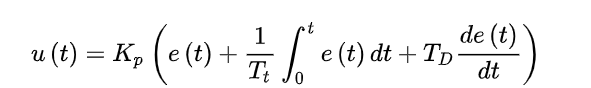Kp——比例增益，Kp与比例度成倒数关系；

Tt——积分时间常数；

TD——微分时间常数；

u（t）——PID的输出信号；

e（t）——给定值r（t）与测量值之差。

PID的增量式算法解析：

由于PID是根据偏差的比例系数，积分系数，微分系数进行调控，首先对三个参数进行一个简单的解释：
比例系数：测量值发生变化时的变化值乘以的系数（输出量与测量值的变化量成比例的系数，与测量值的变化量有关，而非测量值与给定值的差有关）

积分系数：测量值与给定值差乘以的系数（只要测量值与给定值的偏差一直存在，那么迭代过程种积分一直在起作用）

微分系数：这一次测量值差与上一次测量值差的差乘以的系数(用以表征两次测量时变化的剧烈程度)

增量式PID的算法：

/******************** (C) COPYRIGHT  Adrian *************************************
* 文件名  ：PID.c
* 描述    ：增量式PID算法和位置式PID算法
* 版本更新: 2016-05-01
* 硬件连接:
**********************************************************************************/

#include"PID.h"
//采用增量式计算,采样周期为T时间
//Δu(k)=u(k)-u(k-1) = Kp*Δe(k)+ Ki*e(k)+ Kd*[Δe(k)-Δe(k-1)] ，式中Δe(k)=e(k)-e(k-1)
//											= Kp*[e(k)-e(k-1) + (T/Ti)*e(k) + Td*((e(k)-2*e(k-1)+e(k-2))/T)]			(式1-1)
//											= Kp*(1+T/Ti+Td/T)*e(k) - Kp*(1+2*Td/T)*e(k-1) + Kp*Td/T*e(k-2)
//											= A*e(k) - B*e(k-1) + C*e(k-2)																				(式1-2)

//式中:
//					Δu(k)——控制器(也称调节器)的输出增量；
//					e(k)——控制器的输入（常常是设定值与被控量之差，即e(k)=r(k)-c(k)）；
//					Ki=(Kp*T)/Ti 为积分系数
//					Kd=(Kp*Td)/T 为微分系数

//式中:			T:采样周期
//					Kp——控制器的比例放大系数；
//					Ti——控制器的积分时间；
//					Td——控制器的微分时间。

/**
* @file   IncrementalPIDInit
* @brief  增量式PID参数初始化
* @param  *S：PID结构体   Piont：目标值  T:采样周期   Kp：控制器的比例放大系数   Ti：控制器的积分时间；  Td：控制器的微分时间；
* @retval 无
*/
void IncrementalPIDInit(IncrementalPID *S, int32_t Piont, double T, double Kp, double Ti, double Td)
{
S->SetPoint = Piont; 			//目标值
S->SumError = 0;
S->A = Kp*(1+T/Ti+Td/T);	//
S->B = Kp*(1+2*Td/T);	 		//
S->C = Kp*Td/T;	 					//
S->NowError = 0;  				//当前误差
S->LastError = 0; 				//上次误差
S->PrevError = 0;					//上上次误差
}

//Position type PID

/**
* @file   IncrementalPIDCalc(式1-2)
* @brief  增量式PID迭代
* @param  *S：   x：根据反馈量得到的值（属性和Sptr->SetPoint一样）
* @retval PID调节后控制信号增量
*/
int32_t IncrementalPIDCalc(IncrementalPID *Sptr, int32_t x)
{
int32_t Out = 0;

Sptr->NowError = Sptr->SetPoint - x;

Out = Sptr->A * Sptr->NowError - Sptr->B * Sptr->LastError + Sptr->C * Sptr->PrevError; //(式1-2)

Sptr->PrevError = Sptr->LastError;
Sptr->LastError = Sptr->NowError;

return (Out);
}

void PointSetIncrementalPID(IncrementalPID *Sptr, int32_t Piont)//增量式PID设置目标值
{
Sptr->SetPoint = Piont; //目标值
}
void PointSubIncrementalPID(IncrementalPID *Sptr, int32_t Piont)//增量式PID目标值递减
{
Sptr->SetPoint = Sptr->SetPoint - Piont; //目标值
}
void PointAddIncrementalPID(IncrementalPID *Sptr, int32_t Piont)//增量式PID目标值递增
{
Sptr->SetPoint = Sptr->SetPoint + Piont; //目标值
}

//头文件！！！
#ifndef PID_H__
#define PID_H__

#include "stm32f10x.h"

typedef struct IncrementalPID

{
int32_t SetPoint; //设定目标 Desired Value
//	double Proportion; //比例常数 Proportional Const
//	double Integral; 	//积分常数 Integral Const
//	double Derivative; //微分常数 Derivative Const
double A; 	//
double B; 	//
double C; 	//
int32_t NowError;  //Error
int32_t LastError; //Error[-1]
int32_t PrevError; //Error[-2]
int64_t SumError; //误差累计

} IncrementalPID;

void IncrementalPIDInit(IncrementalPID *S, int32_t Piont, double T, double Kp, double Ti, double Td);
int32_t IncrementalPIDCalc(IncrementalPID *Sptr, int32_t x);
void PointSetIncrementalPID(IncrementalPID *Sptr, int32_t Piont);//增量式PID设置目标值

void PointSubIncrementalPID(IncrementalPID *Sptr, int32_t Piont);//增量式PID目标值递减
void PointAddIncrementalPID(IncrementalPID *Sptr, int32_t Piont);//增量式PID目标值递增

// PID结构声明
typedef struct IncrementalPID1
{
int32_t SetPoint; //设定目标 Desired Value
double Kp; //比例常数 Proportional Const
double Ki; //积分常数 Integral Const
double Kd; //微分常数 Derivative Const
int32_t NowError;		//Error
int32_t LastError; //Error[-1]
int32_t PrevError; //Error[-2]
int64_t SumError; //Sums of Errors
}IncrementalPID1;

#endif //PID_H__



展开全文• 在工业应用中 PID 及其衍生算法是应用最广泛的算法之一，是当之无愧的万 能算法，如果能够熟练掌握 PID 算法的设计与实现过程，对于一般的研发人员来 讲，应该是足够应对一般研发问题了，而难能可贵的是，在我所接触...

## 一 PID 算法原理

在工业应用中 PID 及其衍生算法是应用最广泛的算法之一，是当之无愧的万
能算法，如果能够熟练掌握 PID 算法的设计与实现过程，对于一般的研发人员来
讲，应该是足够应对一般研发问题了，而难能可贵的是，在我所接触的控制算法
当中，PID 控制算法又是最简单，最能体现反馈思想的控制算法，可谓经典中的
经典。经典的未必是复杂的，经典的东西常常是简单的，而且是最简单的，想想
牛顿的力学三大定律吧，想想爱因斯坦的质能方程吧，何等的简单！简单的不是
原始的，简单的也不是落后的，简单到了美的程度。先看看 PID 算法的一般形式：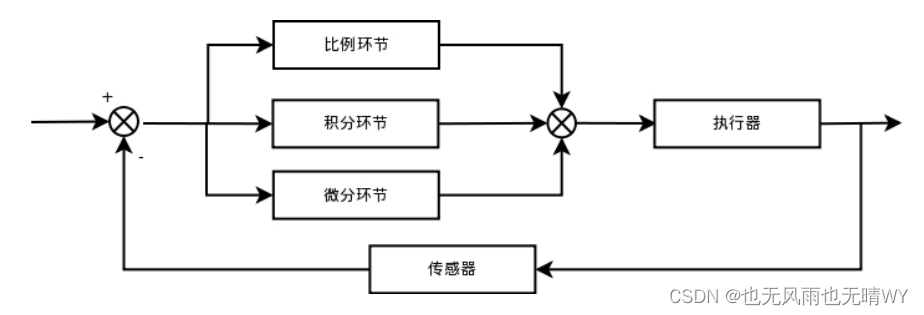PID 的流程简单到了不能再简单的程度，通过误差信号控制被控量，而控制
器本身就是比例、积分、微分三个环节的加和。这里我们规定（在 t 时刻）：

1.输入量为 rin(t);
2.输出量为 rout(t);
3.偏差量为 err(t)=rin(t)-rout(t);


pid 的控制规律为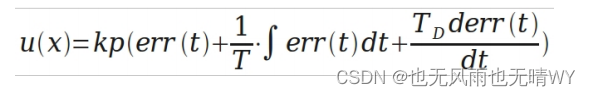理解一下这个公式，主要从下面几个问题着手，为了便于理解，把控制环境
具体一下：

1.规定这个流程是用来为直流电机调速的;
2.输入量 rin(t)为电机转速预定值;
3.输出量 rout(t)为电机转速实际值;
4.执行器为直流电机;
5.传感器为光电码盘，假设码盘为 10 线;
6.直流电机采用 PWM 调速 转速用单位 转/min 表示;


不难看出以下结论：

1.输入量 rin（t）为电机转速预定值（转/min）;
2. 输出量 rout(t)为电机转速实际值（转/min）;
3.偏差量为预定值和实际值之差（转/min）;


那么以下几个问题需要弄清楚：

1.通过 PID 环节之后的 U(t)是什么值呢？
2.控制执行器（直流电机）转动转速应该为电压值（也就是 PWM 占空比）。
3.那么 U(t)与 PWM 之间存在怎样的联系呢？


## 二 PID 算法的离散化

上一节论述了 PID 算法的基本形式，并对其控制过程的实现有了一个
简要的说明，通过上一节的总结，基本已经可以明白 PID 控制的过程。这一节中
先继续上一节内容补充说明一下。

1.说明一下反馈控制的原理，通过上一节的框图不难看出，PID 控制其实是
对偏差的控制过程;
2.如果偏差为 0,则比例环节不起作用，只有存在偏差时，比例环节才起作用。
3.积分环节主要是用来消除静差，所谓静差，就是系统稳定后输出值和设定
值之间的差值，积分环节实际上就是偏差累计的过程，把累计的误差加到原有系
统上以抵消系统造成的静差。
4.而微分信号则反应了偏差信号的变化规律，或者说是变化趋势，根据偏差
信号的变化趋势来进行超前调节，从而增加了系统的快速性。


好了，关于 PID 的基本说明就补充到这里，下面将对 PID 连续系统离散化
从而方便在处理器上实现。下面把连续状态的公式再贴一下：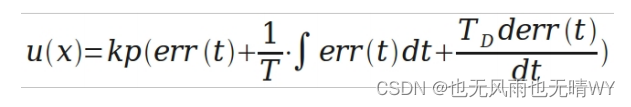假设采样间隔为 T，则在第 K T 时刻

偏差 err(K)=rin(K)-rout(K);
积分环节用加和的形式表示，即 err(K)+err(K+1)+……;
微分环节用斜率的形式表示，即[err(K)-err(K-1)]/T;
从而形成如下 PID 离散表示形式：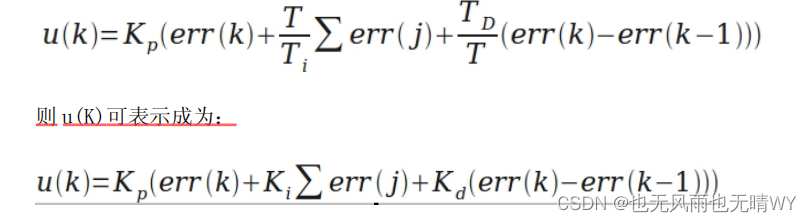至于说 Kp、Ki、Kd 三个参数的具体表达式，我想可以轻松的推出了，这里节省
时间，不再详细表示了。
其实到这里为止，PID 的基本离散表示形式已经出来了。目前的这种表述形式属
于位置型 PID，另外一种表述方式为增量式 PID，由 U 上述表达式可以轻易得到：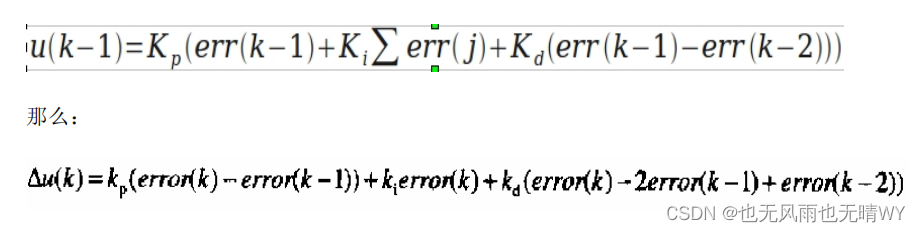这就是离散化 PID 的增量式表示方式，由公式可以看出，增量式的表达结果和最
近三次的偏差有关，这样就大大提高了系统的稳定性。
需要注意的是最终的输出。
结果应该为：

u(K)+增量调节值;
PID 的离散化过程基本思路就是这样，下面是将离散化的公式转换成为 C 语言，
从而实现微控制器的控制作用。


## 三 位置型 PID 的 C 语言实现

上一节中已经抽象出了位置性 PID 和增量型 PID 的数学表达式，这一节，重
点讲解 C 语言代码的实现过程，算法的 C 语言实现过程具有一般性，通过 PID
算法的 C 语言实现，可以以此类推，设计其它算法的 C 语言实现。

第一步：定义 PID 变量结构体，代码如下：

struct _pid{
float SetSpeed; //定义设定值
float ActualSpeed; //定义实际值
float err; //定义偏差值
float err_last; //定义上一个偏差值
float Kp,Ki,Kd; //定义比例、积分、微分系数
float voltage; //定义电压值（控制执行器的变量）
float integral; //定义积分值
}pid;


控制算法中所需要用到的参数在一个结构体中统一定义，方便后面的使用。
第二部：初始化变量，代码如下：

void PID_init(){
printf("PID_init begin \n");
pid.SetSpeed=0.0;
pid.ActualSpeed=0.0;
pid.err=0.0;
pid.err_last=0.0;
pid.voltage=0.0;
pid.integral=0.0;
pid.Kp=0.2;
pid.Ki=0.015;
pid.Kd=0.2;
printf("PID_init end \n");
}


统一初始化变量，尤其是 Kp,Ki,Kd 三个参数，调试过程当中，对于要求的控制
效果，可以通过调节这三个量直接进行调节。

第三步：编写控制算法，代码如下：

float PID_realize(float speed){
pid.SetSpeed=speed;
pid.err=pid.SetSpeed-pid.ActualSpeed;
pid.integral+=pid.err;
pid.voltage=pid.Kp*pid.err+pid.Ki*pid.integral+pid.Kd*(pid.err-pi
d.err_last);
pid.err_last=pid.err;
pid.ActualSpeed=pid.voltage*1.0;
return pid.ActualSpeed;
}


注意：这里用了最基本的算法实现形式，没有考虑死区问题，没有设定上下限，
只是对公式的一种直接的实现，后面的介绍当中还会逐渐的对此改进。
到此为止，PID 的基本实现部分就初步完成了。下面是测试代码：

int main(){
printf("System begin \n");
PID_init();
int count=0;
while(count<1000)
{
float speed=PID_realize(200.0);
printf("%f\n",speed);
count++;
}
return 0;
}


下面是经过 1000 次的调节后输出的 1000 个数据（具体的参数整定过程就不说明
了，网上这种说明非常多）：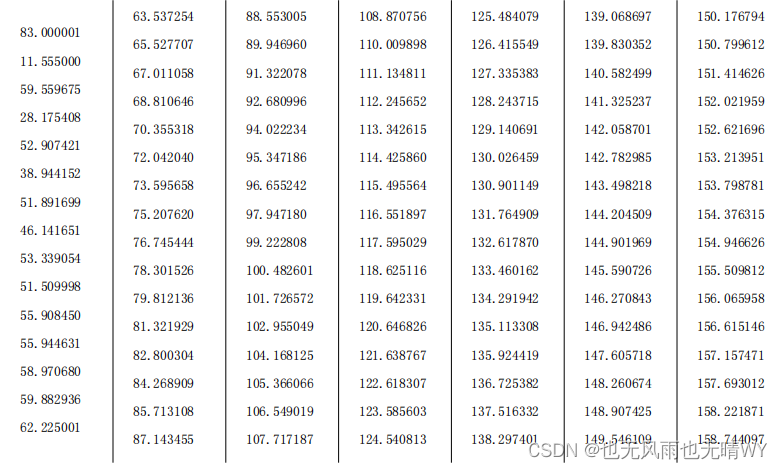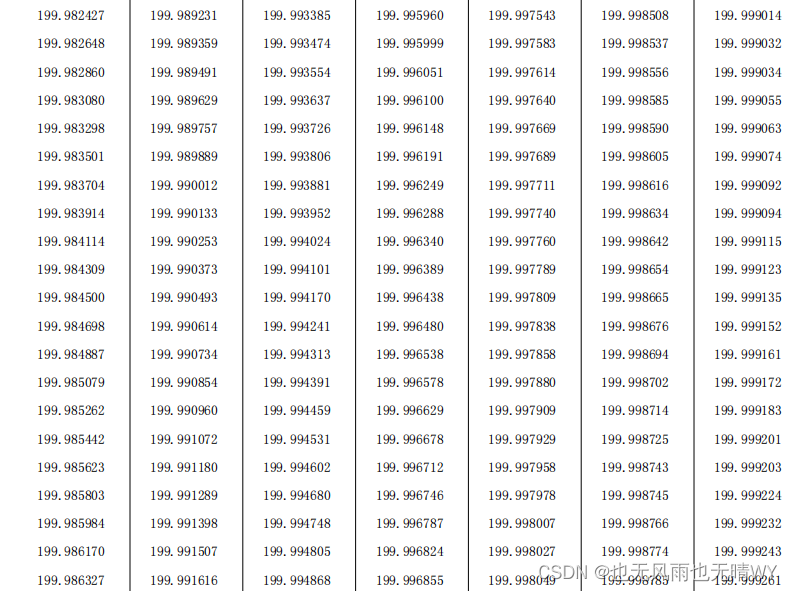## 四 增量型 PID 的 C 语言实现

上一节中介绍了最简单的位置型 PID 的实现手段，这一节主要讲解增量式 PID
的实现方法。实现过程仍然是分为定义变量、初始化变量、实现
控制算法函数、算法测试四个部分，这里直接给出代码了。

#include<stdio.h>
#include<stdlib.h>
struct _pid{
float SetSpeed; //定义设定值
float ActualSpeed; //定义实际值
float err; //定义偏差值
float err_next; //定义上一个偏差值
float err_last; //定义最上前的偏差值
float Kp,Ki,Kd; //定义比例、积分、微分系数
}pid;
void PID_init(){
pid.SetSpeed=0.0;
pid.ActualSpeed=0.0;
pid.err=0.0;
pid.err_last=0.0;
pid.err_next=0.0;
pid.Kp=0.2;
pid.Ki=0.015;
pid.Kd=0.2;
}
float PID_realize(float speed){
pid.SetSpeed=speed;
pid.err=pid.SetSpeed-pid.ActualSpeed;
float
incrementSpeed=pid.Kp*(pid.err-pid.err_next)+pid.Ki*pid.err+pid.Kd*(p
id.err-2*pid.err_next+pid.err_last);
pid.ActualSpeed+=incrementSpeed;
pid.err_last=pid.err_next;
pid.err_next=pid.err;
return pid.ActualSpeed;
}
int main(){
PID_init();
int count=0;
while(count<1000)
{
float speed=PID_realize(200.0);
printf("%f\n",speed);
count++;
}
return 0;
}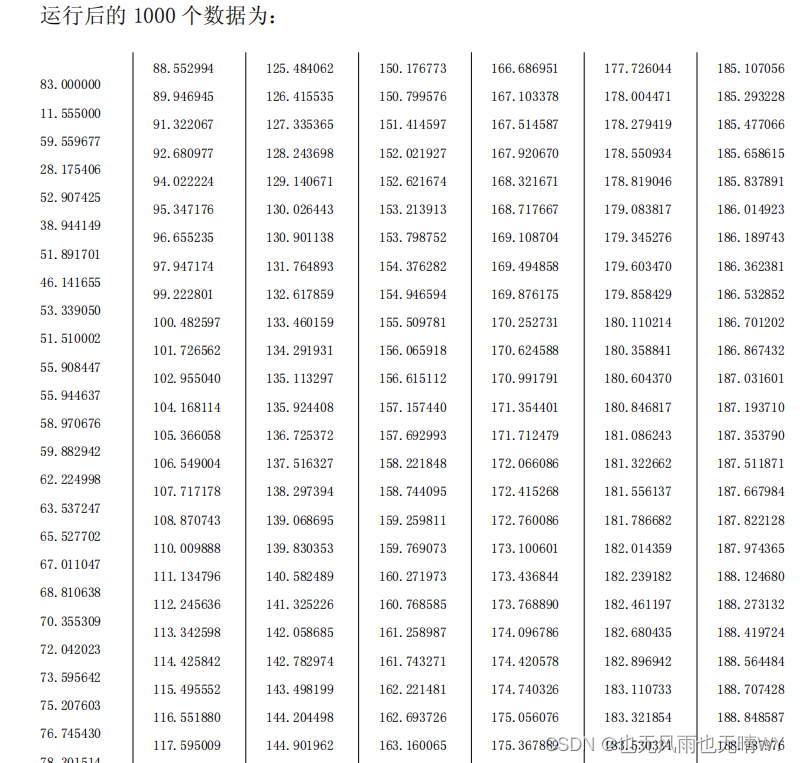## 五 积分分离的 PID 控制算法 C 语言实现

通过前面基本上已经弄清楚了 PID 控制算法的最常规的表达方
法。在普通 PID 控制中，引入积分环节的目的，主要是为了消除静差，提高控制
精度。但是在启动、结束或大幅度增减设定时，短时间内系统输出有很大的偏差，
会造成 PID 运算的积分积累，导致控制量超过执行机构可能允许的最大动作范围
对应极限控制量，从而引起较大的超调，甚至是震荡，这是绝对不允许的

为了克服这一问题，引入了积分分离的概念，其基本思路是 当被控量与设定
值偏差较大时，取消积分作用; 当被控量接近给定值时，引入积分控制，以消除
静差，提高精度。其具体实现代码如下：

pid.Kp=0.2;
pid.Ki=0.04;
pid.Kd=0.2; //初始化过程
if(abs(pid.err)>200)
{
index=0;
}else{
index=1;
pid.integral+=pid.err;
}
pid.voltage=pid.Kp*pid.err+index*pid.Ki*pid.integral+pid.Kd*(pid.
err-pid.err_last); //算法具体实现过程


同样采集 1000 个量，会发现，系统到 199 所有的时间是原来时间的 1/2,系统的快
速性得到了提高。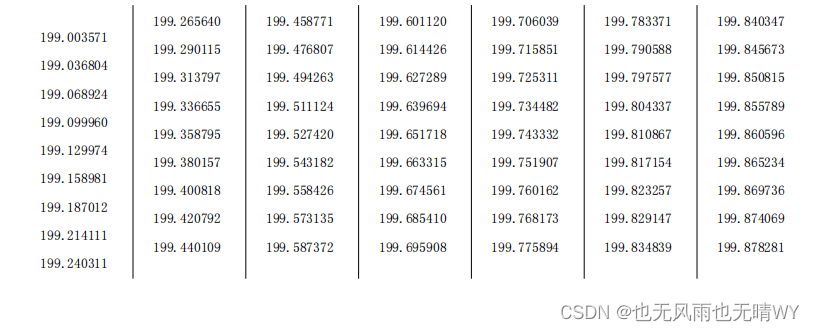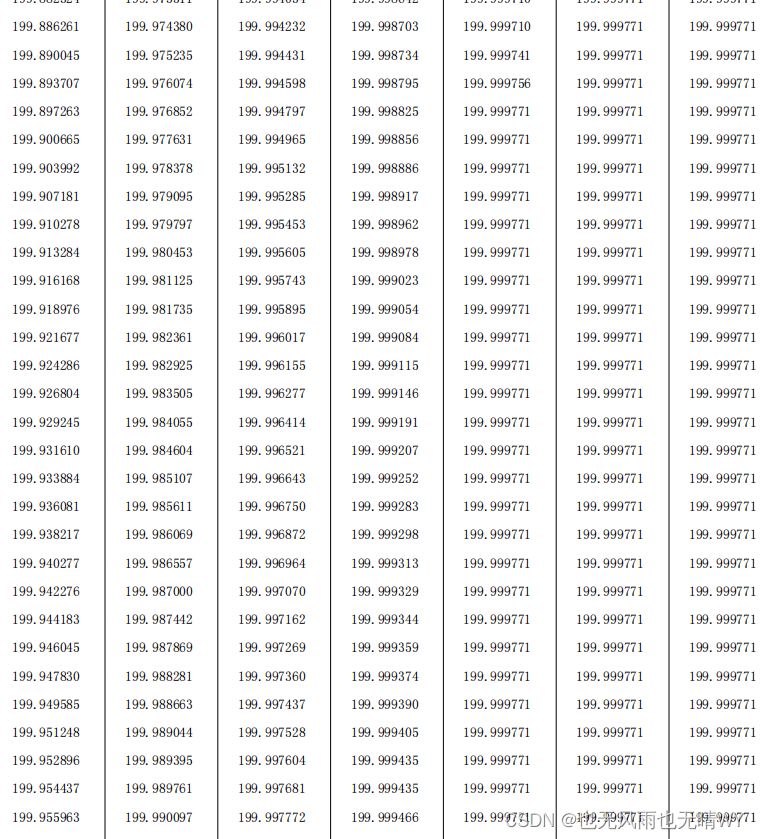## 六 抗积分饱和的 PID 控制算法 C 语言实现

所谓的积分饱和现象是指如果系统存在一个方向的偏差，PID 控制器的输出
由于积分作用的不断累加而加大，从而导致执行机构达到极限位置，若控制器输
出 U(k)继续增大，执行器开度不可能再增大，此时计算机输出控制量超出了正
常运行范围而进入饱和区。一旦系统出现反向偏差，u(k)逐渐从饱和区退出
。进
入饱和区越深则退出饱和区时间越长。在这段时间里，执行机构仍然停留在极限
位置而不随偏差反向而立即做出相应的改变，这时系统就像失控一样，造成控制
性能恶化，这种现象称为积分饱和现象或积分失控现象。

防止积分饱和的方法之一就是抗积分饱和法，该方法的思路是在计算 u(k)
时，首先判断上一时刻的控制量 u(k-1)是否已经超出了极限范围： 如果
u(k-1)>umax，则只累加负偏差; 如果 u(k-1)<umin，则只累加正偏差。从而避
免控制量长时间停留在饱和区。直接贴出代码，不懂的看看前面几节的介绍。

struct _pid{
float SetSpeed; //定义设定值
float ActualSpeed; //定义实际值
float err; //定义偏差值
float err_last; //定义上一个偏差值
float Kp,Ki,Kd; //定义比例、积分、微分系数
float voltage; //定义电压值（控制执行器的变量）
float integral; //定义积分值
float umax;
float umin;
}pid;
void PID_init(){
printf("PID_init begin \n");
pid.SetSpeed=0.0;
pid.ActualSpeed=0.0;
pid.err=0.0;
pid.err_last=0.0;
pid.voltage=0.0;
pid.integral=0.0;
pid.Kp=0.2;
pid.Ki=0.1; //注意，和上几次相比，这里加大了积分环节的值
pid.Kd=0.2;
pid.umax=400;
pid.umin=-200;
printf("PID_init end \n");
}
float PID_realize(float speed){
int index;
PID 控制----C 语言讲解
pid.SetSpeed=speed;
pid.err=pid.SetSpeed-pid.ActualSpeed;
if(pid.ActualSpeed>pid.umax) //灰色底色表示抗积分饱和的实现
{
if(abs(pid.err)>200) //蓝色标注为积分分离过程
{
index=0;
}else{
index=1;
if(pid.err<0)
{
pid.integral+=pid.err;
} }
}else if(pid.ActualSpeed<pid.umin){
if(abs(pid.err)>200) //积分分离过程
{
index=0;
}else{
index=1;
if(pid.err>0)
{
pid.integral+=pid.err;
} }
}else{
if(abs(pid.err)>200) //积分分离过程
{
index=0;
}else{
index=1;
pid.integral+=pid.err;
} }
pid.voltage=pid.Kp*pid.err+index*pid.Ki*pid.integral+pid.Kd*(pid.
err-pid.err_last);
pid.err_last=pid.err;
pid.ActualSpeed=pid.voltage*1.0;
return pid.ActualSpeed;
}


最终的测试程序运算结果如下，可以明显的看出系统的稳定时间相对前几次来讲
缩短了不少。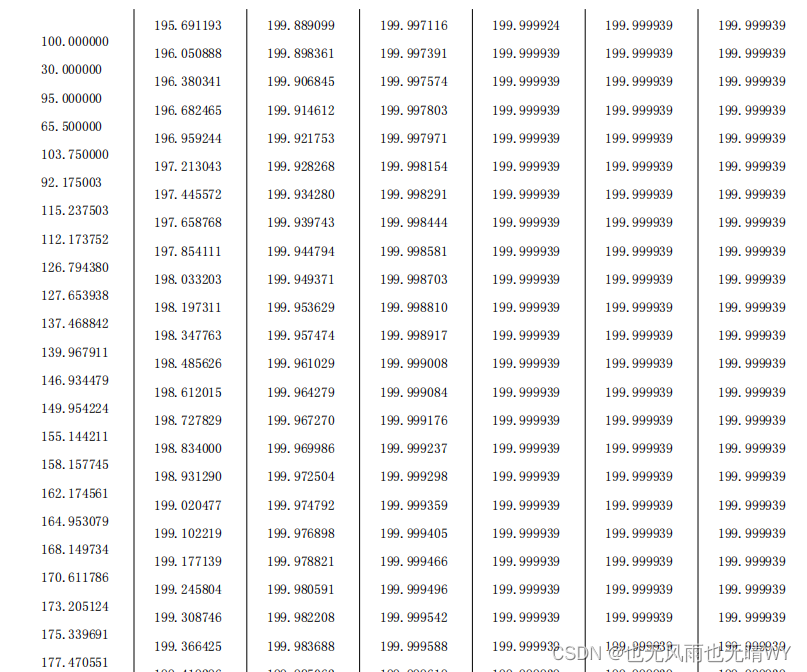就讲到这里了，点个赞再走吧！

展开全文• pid的一些介绍，希望对大家有帮助，这个资源是网上的，分享给大家供参考学习，非商业使用，希望大家一起学习进步
• 增量型PID的离散化公式 △u=u(n)−u(n−1)=Kp*(err(n)−err(n−1))+Ki*err(n)+Kd*(err(n)−2err(n−1)+err(n−2)) err(n)---n时刻的误差采样值（setpoint-input），这里的input也称为反馈值。 err(n-1)---n时刻的...
• PID控制算法的C语言实现四增量型PID的C语言实现[收集].pdf
•PID C语言
•PID类型
• 位置型PID增量PID调节直流电机 一、思路： 无论是位置型还增量型的PID都是为了调节PWM波，使电机的转速达到设定值。这次实验目的主要是实现用机智云app控制电机并使电机的转速达到设定值后保持稳定。 二、实现过程：...
• (1)包含必要的头文件及定义程序需要的结构。 　 　: window._bd_share_config = { "common": { "bdSnsKey": {}, "bdText":
• 学了这么多年的PID，总结一下，准备面试。 1. 公式 u(t)=Kpe(t)+Ki∫0te(t)dt+Kdde(t)dt u(t)=K_p e(t) + K_i \int_0^te(t)dt + K_d \frac{de(t)}{dt} u(t)=Kp​e(t)+Ki​∫0t​e(t)dt+Kd​dtde(t)​ 2. 意义 ...位置型PID 增量型PID
• 增量型PID算法——C语言实现 /* 增量型pid */ #include<stdio.h> #include<stdlib.h> struct _pid{ float SetSpeed; //定义设定值 float ActualSpeed; //定义实际值 float err; //定义偏差值 float...
• 增量型PID的C++语言实现 上一节中介绍了最简单的位置型PID的实现手段，这一节主要讲解增量式PID的实现方法.实现过程仍然是分为定义变量、初始化变量、实现控制算法函数、算法测试四个部分，这里直接给出代码了...PID控制算法
• ## 增量型PID算法

千次阅读 2017-12-11 10:37:50
#include using namespace std; struct _PID { float SetSpeed; float ActualSpeed; float Kp; float Ki; float Kd; float err; float Last_err; float Up_err; float PID_in
• 在STM32上实现增量PID算法前言一、pandas是什么？二、使用步骤1.引入库2.读入数据总结 前言 提示：这里可以添加本文要记录的大概内容： 例如：随着人工智能的不断发展，机器学习这门技术也越来越重要，很多人都...
• 有位置编程可以知道这个编程也很简单，戒指接贴出代码了： #include "stdio.h" struct _pid{ float SetSpeed; float ActualSpeed; float Err; float Err_Last; float Err_Next;  float Kp,Ki,Kd; }...
•增量式PID
• 上一节中介绍了最简单的位置型PID的实现手段，这一节主要讲解增量式PID的实现方法，位置型和增量型PID的数学公式请参见我的系列文《PID控制算法的C语言实现二》中的讲解。实现过程仍然是分为定义变量、初始化变量、...
• 1PID控制算法 什么是PID PID 控制器以各种形式使用超过了 1 世纪，广泛应用在机械设备、气动设备 和电子设备.在工业应用中PID及其衍生算法是应用最广泛的算法之一，是当之无愧的万能算法 PID 实指“比例 ...
• labview PID控制程序 增量PID温度测量，很好用，有说明
• 增量型PID算法汇编程序，增量式PID算法的输出量为 UnΔ = Kp[(en-en-1)+(T/Ti)en+(Td/T)(en-2*en-1+en-2)] 式中，en、en-1、en-2分别为第n次、n-1次和n-2次的偏差值，Kp、Ti、Td分别为比例系数、积分系数和微分...
• AB编码器增量PID控制.rar,AB编码器增量PID控制,Output,bsp_gpio.d,stm32f10x_fsmc.d,anbt_dmp_mpu6050.o,Project.build_log.htm,stm32f10x_dma.d,delay.crf,stm32f10x_rtc.crf,balance.crf,bsp_sys.d,stm32f10x_can....
• IncrementLocation = (*pid).Kp * ((*pid).err_now_0 - (*pid).err_next_1) + (*pid).Ki * (*pid).err_now_0 + (*pid).Kd * ((*pid).err_now_0 - 2 * (*pid).err_next_1 + (*pid).err_last_2); /*△Uo(n) = P * [e...增量型PID
• 本文从数字化实现的角度理解PI（proportional integral, PI）环节，为数字化实现PI打下基础。... 参考文献  PID控制器参数整定方法及其应用研究, 浙江大学硕士, 何芝强, 2005 扩充材料 增量PID控制——百度百科...